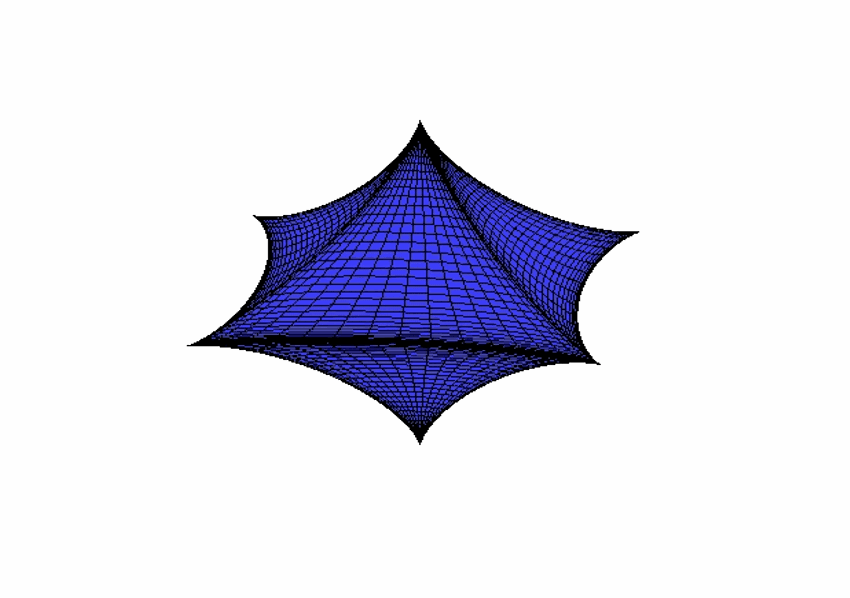# Beveled Cube from a Sphere

This post is about how one can map a sphere to a “rounded”, “smoothed” or as I prefer to call it a “beveled” cube. We will as a bonus also see how to make a inverted cube, in a very simple way using MATLAB.

The reason for doing this is I wanted to create an ordinary cube for an assignment in a visualization course. By doing this simple mapping I’m about to describe, we can actually get a very nice looking smoothed cube if we use a sphere.

In MATLAB we have the function Sphere(n) which creates coordinates for a sphere, given a resolution n, so I figured if I could map a sphere into a cube, hmm…. The reason was, I didn’t want to manually enter the coordinates for the cube, that’s too much work. I have done that in OpenGL before, I have had enough of that (hehe). Additionally if I could get a beveled cube, that would be even better.

The sphere is created using:

[x, y, z] = sphere(n);
h         = surf(x, y, z)


We can then later modify the data using set in the following way:

ex = 2^(-5*i/50);
set(h, 'xdata', abs(x).^ex.*sign(x)/2,...
'ydata', abs(y).^ex.*sign(y)/2,...
'zdata', abs(z).^ex.*sign(z)/2)


In the animation below, I used. The sign is telling the mapping which direction of which axis the coordinates are to be stretched. In the x axis direction, we get two directions1, in y we get two and in z we get two. abs is balancing the stretching, if we don’t have the abs, we would not get a nice mapping.

Consider x=linspace(0,1,500); plot(x, x.^20)

Sincethe mapping will stay in, the same goes for my mapping and this was my first idea. By simply changing the exponent, we would get different shapes of the sphere. I had to take care of the directions using sign and abs otherwise I would get a folded sphere.

The variable that controls the shape is i:
Inverted  i = -15
Octahedron  i = -10
Sphere  i = 0
Beveled Cube i = 50

The animation shown below is stepping through these values of i with increment 1. The animation is wide, so the sphere unfortunately looks like an ellipsoid.If we continue to increase i, choose n to be low enough, we get an ordinary cube. Anyway, comment if you like it 😉